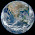### Make A Simple Climate Model From Scratch

I wanted to make a simple climate model from scratch, so I took some of the data that's available and created one. I've written up the process in detail here for anyone who wants to do something similar and have created a simple tool using this that you can play with here.

#### Assumptions

For the time period in question, CO2 levels are enough to roughly predict average global temperature anomaly (anomaly is the current temperature minus the average temperature from a reference period). That's it. That's the only assumption this is based on.

It should be clear from that statement that this isn't a rigorous model. I don't try to factor in ice albedo, aerosol levels, etc. This is basically a curve fitting exercise to crudely estimate how sensitive temperature is to CO2 levels that I found fun and is not a valid scientific way to project temperatures. For better/more robust projections, use something like the CMIP (I use CMIP5 for most of the projections on this site).

#### Data

I just worked with two data sets here:
1. Historic temperature anomaly (taken from Berkeley Earth)
2. Historic CO2 levels (taken from the Institute for Atmospheric and Climate Science and the NOAA)

#### Estimating the relationship between CO2 and temperature anomaly

Since radiative forcing from CO2 is proportional to the log of CO2 levels, I worked with ln(CO2). Since CO2 is assumed to be the driver of temperature increases here, I wanted to estimate the lag between a rise in CO2 and a rise in temperature. To do that, I put ln(CO2) levels on the same scale as temperature anomalies, and plotted both vs time to yield the following plot:

It looks like temperatures rise roughly a decade after CO2 rises. Doing the same thing but shifting the CO2 levels by a decade yields this plot:

They look better aligned. Taking that then, we can plot temperature vs ln(CO2 from a decade ago):

A question then arises. How good are our temperature data sets? The temperature data I linked earlier have the uncertainties associated with them, and they trend down until the last few decades. Arbitrarily taking 0.05 degrees as the uncertainty to stay below, that means temperatures from 1960 on are more accurate. That means we can work with temperatures from 1960 - 2016 and CO2 levels from 1950 - 2006.

Taking the best fit line, we generate a very simple formula relating temperature anomaly and CO2 level. The number in front of the ln(CO2) term is proportional to a term often called 'sensitivity' and it is basically 'the temperature increase that you get when you double CO2 levels'. To get that from this equation requires a bit of math. Calling the number in front of ln(CO2) 'm' , the constant term at the end 'b', anomaly with starting CO2 'anomaly', and anomaly with doubled CO2 'doubled_anomaly':

anomaly = m*ln(CO2) + b
doubled_anomaly = m*ln(2*CO2) + b

We know ln(a*b) = ln(a) + ln(b). Thus:

doubled_anomaly = m*ln(2) + m*ln(CO2) + b

Using the definition of anomaly, we find:

doubled_anomaly = m*ln(2) + anomaly

Thus, doubling CO2 increases the anomaly by m*ln(2). In other words, the 'sensitivity' here is just the number in front of ln(CO2) multiplied by ln(2). For the fit we had above, that gives us ~2.86 and that is the default value in the tool I mentioned at the beginning.

Now...from the plot above that determined our equation, it's clear that a lot of lines will fit reasonably well. The sensitivity could be a lot of different values and still give you reasonable fits. To account for that, I took sensitivities from 2.5 to 3.5, found lines for those, and came up with a number of values for b and sensitivity in the equation above. I noticed that those were roughly linear:

#### Creating the climate model

And that's it...we now have a very crude model for giving us the temperature anomaly for a given CO2 level with a range of different sensitivity values:

How well does it work? I made a tool mentioned above that you can use. As a note, in the tool I wanted to compare with the Paris agreement's target of <1.5 degrees of increase and upper limit of 2 degrees of increase compared with pre-industrial levels. There doesn't seem to be an exact definition of 'pre-industrial' so I took 1850 - 1900 as that time period. Doing so means shifting all anomalies here up by 0.36 degrees so that the 1850-1900 average is an anomaly of 0. An example screenshot with it is here:

1.How does value of "Relative Concentration Pathways" or rcp fit into your equation?

I used System Dynamics over 17 years ago to build stock and flow models that align the cause and effect of Greenhouse Gas effect with actual data over prior years. Then use the model to develop foresight about sensitivity of global temperature to changes in CO2. I had a website setup but took it down. Maybe I should redo my work and post it. I will use Insight Maker https://insightmaker.com

Your conclusion is correct that there is a ten year delay between CO2 emissions and temperature increases. There are feedback loops within the layers of the atmosphere that also create 20 and 30 year delay loops.

2.The RCPs don't fit into it. RCPs are future emission scenarios.

I'd be interested in seeing your model when you post it. I looked for really simple ones online before making this and didn't find any and it was pretty fun to go through.